Believe it or not

Nimit Theeraleekul B. Eng. (Communication)

(1 February 2012)

It is interesting to note that despite of worldwide using mobile phones, scientists still do not clearly understand how they communicate with each other. Nowadays modern physicists explain that mobile phones connect with each other via radio wave - that okay, but the problem - it is a magic wave which is not natural and understandable one! Armed with a new concept of the mechanism of vacuum medium, then it is not difficult to explain how it works. Here is the short story, while more detail could be seen in the paper “Completed Maxwell electromagnetic field theory” presenting in www.vacuum-mechanics.com.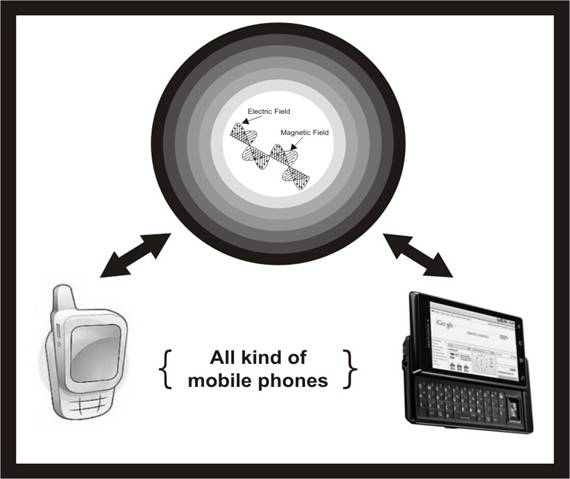Figure 1. Magic wave used for mobile phones communication.

1) Today it is well known that all kind of mobile phones is workable by using radio wave communication system. Anyway, the problem is that modern physicists cannot clearly explain how radio wave could be generated, radiate and propagate (travel) via vacuum space! What we were told is that radio wave is a special kind of wave which could work without using any medium as the carrier (such as air was used as the carrier for sound wave); it seems that radio wave is something like a magic wave!

But the problem is why radio wave was thought to be a special kind of wave - which needs no medium for propagation? This is contrast to all other kinds of natural wave, which according to physics (science of the nature) wave was the action of the vibrations of some physical medium such as water wave was the vibration of the water.

2) Actually the reason that radio wave was thought to work without using any medium, it is because of the consequence of Einstein special theory of relativity(SRT), which based on the concept that vacuum medium is an empty space (without any physical thing). And according to STR, the famous Michelson-Morley experiment was used to prove that the aether is not existed!

Anyway, we have shown (in the paper “Einstein relativity vs. Aether theory”) that it is obvious prove that the aether is existed! Also we have point out (in the paper “What is curved space, could we understand it?”) that the result of Michelson-Morley experiment was misinterpreted (as there is no aether drags along the earth). The reason is because that Einstein general theory of relativity has verified that there is aether drags along the rotating earth!

3) Now let us talk about (what which we was told) how radio wave could travel without using any medium. Originally according to Maxwell electromagnetic field theory in which J.C. Maxwell has developed it, aether was used in the theory, so there is no problem about the wave propagation.

Without aether, modern physicists have to find a new way for the wave propagation. And what they said is that radio wave, i.e. electromagnetic wave, could propagation by itself because there is mutual creation between electric and magnetic field. But the problem is that electric and magnetic field are simultaneously created (at the same time), then how could they create each other, because it will violate the principle of causality!

4) Armed with the new concept of vacuum medium, which is not the same thing as the aether that filled empty vacuum space; instead it is the fabric structure of space-time itself, i.e. vacuum space was built from vacuum medium! And based on the new concept of vacuum mechanics (mechanism of vacuum medium), we can derive the theory of Maxwell and then it is not difficult to understand how electromagnetic wave work as follow.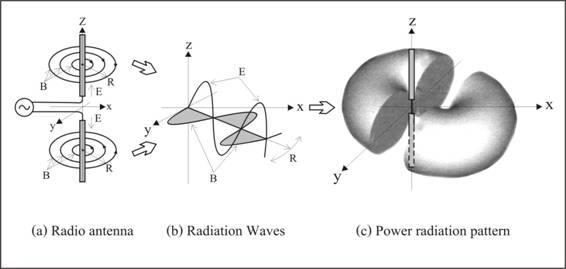Figure 2. A realistic radio wave for mobile phones communication.

According to diagram fig.2 (a), radio signal was generated from mobile phone antenna via the action of stress–strain relation in vacuum medium. The generated electromagnetic wave as show in 2 (b) will radiate as a donut shape pattern around the phone as show in (c), which is then expand to the other nearby mobile phone for receiving the signal. In the reverse way, at the same time, the receiving phone is also sending his signal to the first phone; this is a two- way communication which enables us to talk with our friend simultaneously.

5) Now we will talk about the interaction mechanism between charge particles; conventionally different type of charge particles attract, while the same type ones repel, but there is no explanation why? Armed with the concept that proton and electron are condensed vacuum medium, which act like tiny black holes, and then it is easy to understand that different size of charge particles attract, while the same sizes repel as below.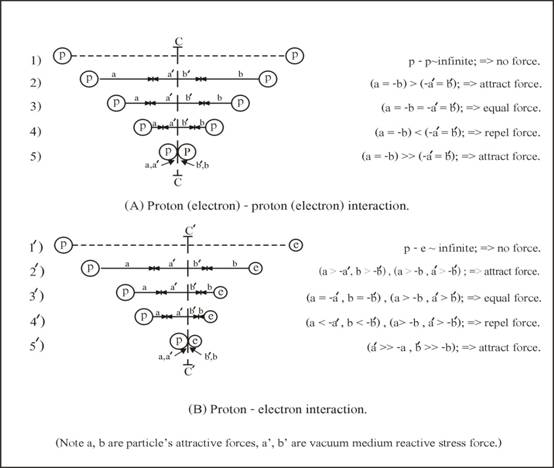Figure 3. Interaction mechanisms between charges particles.

According to diagram in fig. 3(A) it is the interaction between proton and proton or between electron and electron. For case (1) when the separate distance is infinity, no interaction force between them is appeared. Case (2) when they are closing together, they begin to attract each other because both of them are tiny black holes. Case (3) at a certain distance where the attractive forces of the black holes is equal to the repulsive force of the internal stress in vacuum medium between them (because black holes create internal stress in the vacuum medium around), then they stop to attract each other. Cases (4) when the separated distance is closer than in (3), then they repel each other. This is the phenomenon we are familiar - same type of charge repel! Case (5) when both particles are forced to stick together, that is the attract force is greater than the reaction force of the internal stress in vacuum medium.

(Note that the vertical line c – c is perpendicular and divide half the line joint between p and p, while the line c’ – c’ in (B) is closed to e than p due to their different size.)

In fig. 3(B), it is the interaction between proton and electron. Case (1’) and (2’) are the same situation as (1) and (2) in (A). Case (3’) is the same as in (3) (A), only the separate distance between p and e is smaller than in (3) (A). This is because of the different size between proton and electron. And this is the phenomenon we are familiar - different type of charge attract. Case (4’), when the separated distance is closer than in (3’), they begin to repel each other as in case (3) (A). Case (5’) it is the same situation as (5) in (A).

6) Now let us use our new concept of vacuum mechanics to solve the problem about light wave packet (photon). Conventionally we have learnt that it was generated from an exited atom, in which an electron in an excited state (of higher energy) jump back to normal state (of lower energy) as show in diagram fig.4 (a). But the problem - we do not know what the photon is, how it was created and why its speed is constant!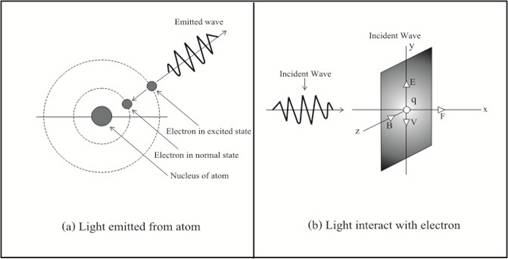Figure 4. Mechanism of light waves.

The crucial point of the photon’s problem is that conventionally vacuum space is empty, so inside and outside atom is void! Instead, if we adopt our new concept that atom was immerged in the sea of vacuum medium, then it not difficult to solve the problem as follow.

In diagram fig. 5(b), suppose light is coming from a source and is acting on an electron charge q with its electric field E component and driving it up and down with velocity v. Then magnetic field B component (which is right angle to the electric field E) will act on the moving electron and create the resultant force F. This driving force F is in the direction of the propagation of light and was called “radiation pressure” or light pressure!

Now return to explain how light is emitted from an atom by using vacuum medium wave packet. In diagram fig. 5(a), when an electron was hit by a photon which its energy is big enough, it will moves from normal state of lower energy to excited state of higher energy. After the collision, the exited electron will jump back from unstable excited state to its normal lower stable state immediately. The reason is because the “transition time” is very short (about 1.6 nanosecond), then the jumping back electron will transfer its impulse force’s energy to the surrounding vacuum medium. This makes the disturbed vacuum medium oscillates and radiate out as a photon. This is the reverse process of diagram fig. 5(b) mentioned in the previous paragraph.

7) Next let us answer the problem why light speed is constant, in which nowadays there is no explanation why it is be so! Again, we could understand it by using the mechanism of vacuum medium – vacuum mechanics as follow.

For one whom familiar with the velocity of light formula in the empty vacuum space will know that it was as (1)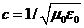………. (1)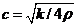………. (2)

(Where,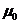= permeability and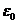= permittivity, while k and ρ are twist modulus and density of vacuum medium.)

And we knew from Maxwell electromagnetic field theory that bothandare empirical constant. But both of them are just the proportional constant that occurred in Maxwell equation, in which it does not tell us why they must be constant!

Instead, according to the concept of vacuum medium which was assumed that it is isotopic and homogenous, so both k and ρ are constant. Then what follow from (2), is that the velocity of light wave c must also be constant! This is the same thing as the velocity of other natural wave such as sound wave for example, which is constant due to the uniform density of the medium air.

Note, we often heard that vacuum medium should be very rigid for creating such a huge speed c of light! This idea comes from the concept that sound speed in rigid medium such as steel which is greater than the non-rigid medium such as air, for example. But this is not quite right; the better method is to compare the same kind of medium such as normal air and hydrogen. For air’s mass density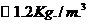which has sound speed 331m/s, while hydrogen mass density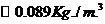has sound speed 1284m/s. The reason is because mass density of hydrogen is lighter than the air (assumed both have the same modulus), so its sound speed is higher than air according to wave speed formula (2) which equal to square root of modulus over density. And because vacuum medium’s density is very dilute, so it is not surprise that light wave speed in vacuum medium must be very high speed!

8) What is photon rest mass? Again this is another problem of photon which was arisen because modern physicists do not accept that light (photon) is the wave packet of vacuum medium.  Armed with our new concept, it is easy to understand the reason why photon has no rest mass while it cannot stop from traveling (propagation) with speed c.

As mentioned early that all kind of natural wave is the vibration of some kind of physical medium, for example sound wave is the vibration of air. And it is easy to understand that a pulse of sound wave (analogous to photon) also has no rest mass too. The reason is because the vibration of air create sound wave that traveling from its source; staying still of sound wave means no vibration or no sound wave (then no rest mass exist). Indeed, all other kind of natural wave such as water wave, including light wave (photon) cannot stop (no vibration) and has no rest mass!

9) Finally we will talk about Poynting’s vector, which is one most important concept of flowing of electromagnetic field energy. Under the conventional concept, there are some crazy problems that involved with it which could be seen from the diagram fig.5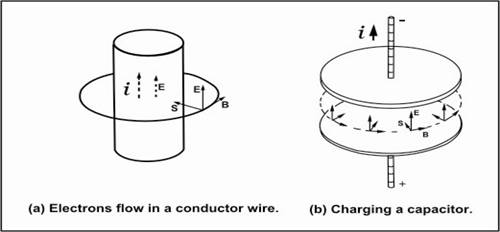Figure 5. Problems involved Poynting’s vector.

In diagram fig. 5(a), according to the convention Poynting vector, it says that heat dissipates energy in a conductor wire is not come from the flow-in electrons current; instead it comes from the field energy around the wire. Another example is the charging energy to store in a capacitor as show in diagram fig. 5(b), it is not come from the flow- in electrons current; but it comes from the field energy around the capacitor. These problems arise because of the ambiguity of the field, i.e. we do not know what really electromagnetic field is!

To solve the problem of the conductor wire, it is easy by using our new concept that electrons are tiny black holes. Because the electrons will attract the surrounding vacuum medium and creating compressive rotational stress in the medium, which is then manifest as electromagnetic energy around the wire. And according to the compressive rotational stress, its direction is point toward into the wire, not outward as the conventional interpretation. In conclusion, the flow-in current (of electrons) give rise to the dissipate heat energy while create the surrounding electromagnetic energy around the wire!

Next to solve the problem of the capacitor; again we will use our new concept that electrons are tiny black holes in the same way as was done with the conductor wire. When the flow-in current of electrons accumulates in the upper disc plate of the capacitor, they will create something like a rotating disc of tiny black holes. Then the rotating disc will drag and rotate the vacuum medium between the two plates and forming a compressive rotational stress in the medium, in which it appears as the flow-in electromagnetic field energy with its (flow) direction as show in the diagram.

In conclusion, the flow-in current give rise to the store energy in the capacitor, while creates a compressive rotational stress in the vacuum medium between the two plates which  appeared as if it is the flow-in electromagnetic field energy into the capacitor!

……………………………..